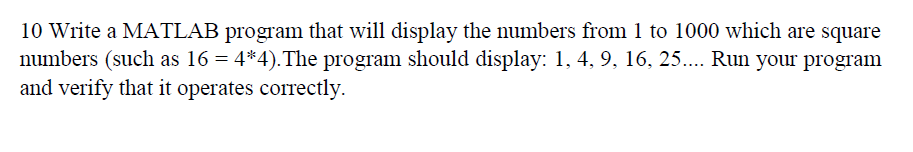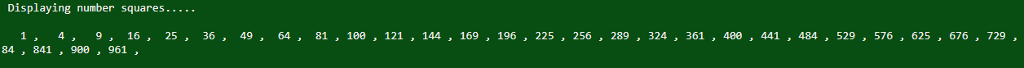# Question & Answer: Write a MATLAB program that will display the numbers from 1 to 100…..Write a MATLAB program that will display the numbers from 1 to 1000 which are square numbers (such as 16 = 4*4).The program should display: 1, 4, 9, 16, 25…. Run your program and verify that it operates correctly.

Don't use plagiarized sources. Get Your Custom Essay on
Question & Answer: Write a MATLAB program that will display the numbers from 1 to 100…..
GET AN ESSAY WRITTEN FOR YOU FROM AS LOW AS \$13/PAGE

% initialize a loop variable i to 1 %
i=1;
% calculate i square and store in res %
res = i * i;
% print the message %
display(‘ Displaying number squares…..’)
% iterate a while loop as long as the res is less than 1000 %
while(res<=1000)
% print the res value %
fprintf(‘%4d ,’,res);
% increment loop variable by 1 %
i = i+1;
% compute i square and store it in res %
res=i*i;
end
display(”);

output: